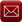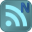You have downloaded this file 0 times in the last 24 hours, limit is 100.

## Consistent units.xls This location is for Registered Users Only.Perhaps you need to login or register.

Description:

Defines consistent units systems for calculations and conversion factors to change between SI and other consistent unit systems.

The easiest set of consistent units is the SI system of metres (meters), kilograms and seconds (MKS). These derive associated units that are commonly used by designers/engineers/analysts, such as Newtons, Joules, Watts, Pascals, plus generically titled items such as density, acceleration, velocity, viscosity and many more. The important point about using consistent units is the necessity to stick with units that work correctly together - not to mix units that do not have a correct relationship with each other.

For example, if using the basic SI units of metres, kilograms and seconds, then:
- Density must be in kg/m3;
- Acceleration must be in metres/sec2;
- Force must be in Newtons, because a Newton is the force it takes to accelerate 1kg at the rate of 1m/sec2. (Force = mass x acceleration, ignoring relativity effects of course), and
- Power must be in Watts (Newtons x metres) per second.

Many engineering companies instruct their engineers to use basic SI units so there are no questions about unit conversion or consistent units. However, this has its downsides, particularly when using FEA. Many people find the use of metres inconvenient, and so the use of millimetres (millimeters) by CAD designers is very popular. If you make the popular choice of millimetres, and prefer using Newtons for force, then this has specific consequences in your use of all other units to preserve consistency. The obvious consequences of choosing Newtons and millimetres are:
- Applied Pressures are Newtons / mm2, which is MegaPascals (MPa)
- Stress results are thus also MPa.
- Young’s Modulus is also in units of pressure, and is thus also MPa.

What is less obvious is that in choosing millimetres and Newtons, you have also forced a change in your mass units. This is because F=ma, and so the question which needs to be asked is: "What mass accelerates at 1mm/sec2 when a force of 1N is applied?" 1mm/sec2 is very slow (1000 times less than 1m/sec2, of course) - the answer is 1 Tonne. Therefore, density is in Tonnes per cubic mm - thus 1e-9 for water and 7.8e-9 for a typical steel. So, if you are running an analysis where gravity is involved (having chosen millimetres and Newtons), then the magnitude of acceleration is 9810 (mm/sec2), point masses are Tonnes and density is in Tonnes / mm3. Additional consequences of choosing millimetres and Newtons occur for heat transfer:
- Conductivity in standard SI: Watts per (metre x Kelvin)
-> Conductivity in your consistent units: milliWatts per (millimetre x Kelvin). (Therefore no change in the numeric value input)
- Heat Flux in Standard SI: Watts per square metre
-> Heat Flux in your consistent units: milliWatts per square millimetre. Therefore numeric input would be reduced by a factor of 1000 compared to Standard SI.
- The same conversion is required for a convection coefficient - ie. W/m2K becomes mW/mm2K so numeric input reduces by a factor of 1000.
- Specific Heat in Standard SI: Joules per (kilogram x Kelvin)
-> Specific Heat in your consistent units: milliJoules per (Tonne x Kelvin). Therefore numeric input would be increased by a factor of 1e6 compared to Standard SI. eg. 4180 [J/kgK] becomes 4.18e9 [mJ/TK]
Note that results such as heat flux will obviously be presented in the same consistent units you have chosen to apply. Imperial Units For those of you who use Imperial Units, you have our sincerest condolences, however the same set of principles apply. A consistent set of units must be used in order to produce a valid analysis. For engineering, a basic set of imperial units is slug (mass), foot (length) and seconds (time). It is critical to note that a pound is not a unit of mass for engineers and should not be used as a unit of mass. A pound is a unit of force. A pound is the unit of force required to accelerate a slug at "1g", which is 32.18 feet/sec2. As most of us do not size each other up by estimating a person's "weight" in slugs, it is thus useful to know that a mass of one slug weighs about 32 pounds force on earth (from the definition above). Again, for many people, the use of feet for length is inconvenient, so inches are often used instead, which thus means some conversions are required to preserve a consistent set of units. The obvious consequence of using inches is that applied pressures, stress results and Youngs Modulus are all in psi (pounds per square inch). This is common, as many sources supply material constants in these units. Not so obvious is that mass is now in units of "12 x slugs". Although some have named this mass unit the "slinch" or "blob", we prefer the colloquially applied name, the "snail". (Thanks to Dr Tim Coates of GKN Aerospace for supplying this useful name for the unit - we are unsure as to whether the term has earlier origins).

Thus density must be in units of snails per cubic inch, which for a typical steel would be 7.3e-4. A snail weighs about 386 pounds force on earth (it is a large snail). If doing thermal analysis using Imperial Units (in inches), then consistent units could be applied by using:
- inch pounds for Work
- inch pounds per second for Power
- (inch pounds per second) per square inch for Heat Flux
- inch pounds per (snail x degree) for Specific Heat.
As most texts do not conveniently supply material constants or coefficients or equations using these units (even densities are often misquoted as weight densities), then some additional conversion and care is required. BTU is a commonly used unit which is the basis of other derived thermal units, and so conversion from BTU style units to those above can be used. It is also possible to use hybrid units (ie. Imperial for mechanical analysis and metric for thermal analysis) in the same model, but extreme caution must be used when doing so. The numerous warnings required for the various analysis systems when using hybrid units are beyond the scope of this article. Our recommendations:
1. ALWAYS use consistent units. That means the base units of Mass, Length and Time must comply with any/all derived associated units you are using in your analysis (such as pressure, force, velocity, density, acceleration etc.).
2. Use metric if you can - the availability of material data and constants in workable imperial units (particularly in thermal and fluids analysis) typically requires arduous conversion to maintain a consistent set of units for analysis. However, units conversion of any sort is best minimised, so you do not become the person blamed for losing the next Mars Climate Orbiter!

Submitted By:
Submitted On:
04 Jun 2009
File Size:
25.00 Kb
415
File Version:
1.3
Rating:Total Votes:5
If you use the formula F=ma then it works provided that the units are consistent. So for the SI system mass will be in kilograms acceleration in meters per second per second and the force is calculated in Newtons. The spreadsheet shows you that you could use the CGS system when mass will be in grams acceleration in centimetres per second per second and the force is calculated in dynes.
Conversion factors from SI to other systems of units are also included.
does not compute.
ashish 12 years ago
Same comments as eskelsev. Probably, one can use it in database linking for some unit conversion in a spreadsheet using vlookup command.
ashish 12 years ago
Same comments as eskelsev. Probably, one can use it in database linking for some unit conversion in a spreadsheet using vlookup command.
eskelsev 12 years ago
Not sure of its usefulness. I could print it out once and never look at it again. Why would I have a spreadsheet?
eskelsev 12 years ago
Not sure of its usefulness. I could print it out once and never look at it again. Why would I have a spreadsheet?

We have 585 guests and 4 members onlineEmail (preferred method of contact)US +1 617 5008224EU +44 113 8152220